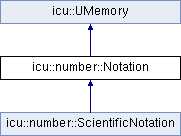ICU 72.1 72.1
icu::number::Notation Class Reference

A class that defines the notation style to be used when formatting numbers in NumberFormatter. More...

`#include <numberformatter.h>`

Inheritance diagram for icu::number::Notation:## Static Public Member Functions

static ScientificNotation scientific ()
Print the number using scientific notation (also known as scientific form, standard index form, or standard form in the UK). More...

static ScientificNotation engineering ()
Print the number using engineering notation, a variant of scientific notation in which the exponent must be divisible by 3. More...

static CompactNotation compactShort ()
Print the number using short-form compact notation. More...

static CompactNotation compactLong ()
Print the number using long-form compact notation. More...

static SimpleNotation simple ()
Print the number using simple notation without any scaling by powers of ten. More...

## Friends

struct impl::MacroProps

class ScientificNotation

class impl::NumberFormatterImpl

class impl::ScientificModifier

class impl::ScientificHandler

class impl::GeneratorHelpers

## Detailed Description

A class that defines the notation style to be used when formatting numbers in NumberFormatter.

Stable:
ICU 60

Definition at line 199 of file numberformatter.h.

## ◆ compactLong()

 static CompactNotation icu::number::Notation::compactLong ( )
static

Print the number using long-form compact notation.

For more information on compact notation, see `compactShort`.

In long form, the powers of ten are spelled out fully. Example outputs in en-US when printing 8.765E7 through 8.765E0:

```88 million
8.8 million
876 thousand
88 thousand
8.8 thousand
876
88
8.8
```
Returns
A CompactNotation for passing to the NumberFormatter notation() setter.
Stable:
ICU 60

## ◆ compactShort()

 static CompactNotation icu::number::Notation::compactShort ( )
static

Print the number using short-form compact notation.

Compact notation, defined in Unicode Technical Standard #35 Part 3 Section 2.4.1, prints numbers with localized prefixes or suffixes corresponding to different powers of ten. Compact notation is similar to engineering notation in how it scales numbers.

Compact notation is ideal for displaying large numbers (over ~1000) to humans while at the same time minimizing screen real estate.

In short form, the powers of ten are abbreviated. In en-US, the abbreviations are "K" for thousands, "M" for millions, "B" for billions, and "T" for trillions. Example outputs in en-US when printing 8.765E7 through 8.765E0:

```88M
8.8M
876K
88K
8.8K
876
88
8.8
```

When compact notation is specified without an explicit rounding precision, numbers are rounded off to the closest integer after scaling the number by the corresponding power of 10, but with a digit shown after the decimal separator if there is only one digit before the decimal separator. The default compact notation rounding precision is equivalent to:

```Precision::integer().withMinDigits(2)
```
Returns
A CompactNotation for passing to the NumberFormatter notation() setter.
Stable:
ICU 60

## ◆ engineering()

 static ScientificNotation icu::number::Notation::engineering ( )
static

Print the number using engineering notation, a variant of scientific notation in which the exponent must be divisible by 3.

Example outputs in en-US when printing 8.765E4 through 8.765E-3:

```87.65E3
8.765E3
876.5E0
87.65E0
8.765E0
876.5E-3
87.65E-3
8.765E-3
0E0
```
Returns
A ScientificNotation for chaining or passing to the NumberFormatter notation() setter.
Stable:
ICU 60

## ◆ scientific()

 static ScientificNotation icu::number::Notation::scientific ( )
static

Print the number using scientific notation (also known as scientific form, standard index form, or standard form in the UK).

The format for scientific notation varies by locale; for example, many Western locales display the number in the form "#E0", where the number is displayed with one digit before the decimal separator, zero or more digits after the decimal separator, and the corresponding power of 10 displayed after the "E".

Example outputs in en-US when printing 8.765E4 through 8.765E-3:

```8.765E4
8.765E3
8.765E2
8.765E1
8.765E0
8.765E-1
8.765E-2
8.765E-3
0E0
```
Returns
A ScientificNotation for chaining or passing to the NumberFormatter notation() setter.
Stable:
ICU 60

## ◆ simple()

 static SimpleNotation icu::number::Notation::simple ( )
static

Print the number using simple notation without any scaling by powers of ten.

This is the default behavior.

Since this is the default behavior, this method needs to be called only when it is necessary to override a previous setting.

Example outputs in en-US when printing 8.765E7 through 8.765E0:

```87,650,000
8,765,000
876,500
87,650
8,765
876.5
87.65
8.765
```
Returns
A SimpleNotation for passing to the NumberFormatter notation() setter.
Stable:
ICU 60

## ◆ impl::GeneratorHelpers

 friend class impl::GeneratorHelpers
friend

Definition at line 398 of file numberformatter.h.

## ◆ impl::MacroProps

 friend struct impl::MacroProps
friend

Definition at line 389 of file numberformatter.h.

## ◆ impl::NumberFormatterImpl

 friend class impl::NumberFormatterImpl
friend

Definition at line 393 of file numberformatter.h.

## ◆ impl::ScientificHandler

 friend class impl::ScientificHandler
friend

Definition at line 395 of file numberformatter.h.

## ◆ impl::ScientificModifier

 friend class impl::ScientificModifier
friend

Definition at line 394 of file numberformatter.h.

## ◆ ScientificNotation

 friend class ScientificNotation
friend

Definition at line 390 of file numberformatter.h.

The documentation for this class was generated from the following file: